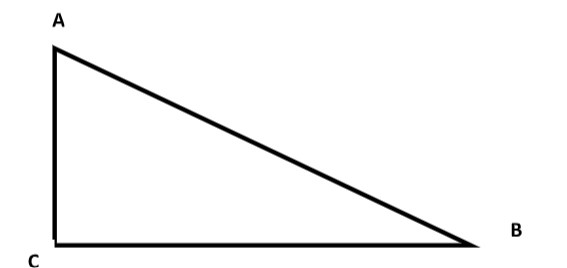QuestionAnswers

# ABC is an isosceles triangle with AC = BC . If $A{B^{2\;}} = {\text{ }}2{\text{ }}A{C^2}$. Prove that ABC is a right angle triangleHint:Because angles opposite equal sides are themselves equal, an isosceles triangle has two equal angles (the ones opposite the two equal sides). Thus, given two equal sides and a single angle, the entire structure of the triangle can be determined.
The Pythagorean equation relates the sides of a right triangle in a simple way, so that if the lengths of any two sides are known the length of the third side can be found. Another corollary of the theorem is that in any right triangle, the hypotenuse is greater than any one of the other sides, but less than their sum.

Complete Step by step Solution:
Given:
AC = BC
ABC is an isosceles triangle
$A{B^{2\;}} = {\text{ }}2{\text{ }}A{C^2}$

To prove: ABC is a right angled triangle

Proof:Consider a right angled triangle ABC as shown in the figure
For ABC to be a right triangle, it should satisfy Pythagoras theorem
i.e $A{B^{2\;}} = {\text{ }}A{C^{2\;}} + {\text{ A}}{C^2} \\ \\$ $A{B^{2\;}} = {\text{ }}A{C^{2\;}} + {\text{ }}B{C^2}$
Now, AC = BC (given)………………(1)
On substitution, we find
$\Rightarrow$$A{B^{2\;}} = {\text{ }}2{\text{ }}A{C^2}$
$\Rightarrow$$A{B^{2\;}} = {\text{ }}A{C^{2\;}} + {\text{ A}}{C^2}$
$\Rightarrow$$A{B^{2\;}} = {\text{ }}A{C^{2\;}} + {\text{ }}B{C^2}$
Here AB is the largest side ,i.e Hypotenuse of triangle ABC.
So, the given sides form Pythagorean triplets.
Hence ABC to be a right triangle right angled at C.

Note:Students should keep in mind the formula of Pythagoras theorem and its implementation on right angled triangles.

Isosceles Triangle FormulaRight Angled Triangle ConstructionsScalene Triangle FormulaCBSE Class 7 Maths Chapter 6 - Triangle and Its Properties FormulasArea of Triangle FormulaArea of Equilateral Triangle FormulaCBSE Class 10 Maths Chapter 2 - Polynomials FormulaTypes of AC MotorsPhasor Representation AcClass 10 IMO Maths Olympiad Sample Question Paper - 2Important Questions for CBSE Class 10 Maths Chapter 2 - PolynomialsImportant Questions for CBSE Class 7 English An Alien Hand Chapter 10 - An Alien HandImportant Questions for CBSE Class 10 Maths & Science with AnswersImportant Questions for CBSE Class 10 Science Chapter 2 - Acids, Bases and SaltsImportant Questions for CBSE Class 10 Maths, Chapter wise Questions with AnswersImportant Questions for CBSE Class 7 English An Alien Hand Chapter 2 - Bringing Up KariImportant Questions for CBSE Class 9 Science Chapter 2 - Is Matter Around Us PureImportant Questions for CBSE Class 6 English A Pact with The Sun Chapter 2 - The Friendly MongooseImportant Questions for CBSE Class 7 English An Alien HandCBSE Previous Year Question Papers Class 10 Maths with SolutionsCBSE Class 10 Maths Question Paper 2019 with Solutions - Free PDFCBSE Class 10 English Communicative Question Paper with SolutionsCBSE Class 10 Hindi A Question Paper with SolutionsCBSE Class 10 Hindi B Question Paper with SolutionsCBSE Class 10 Maths Question Paper 2017CBSE Class 10 Maths Question Paper 2020CBSE Class 10 Science Board Question Paper 2019 with SolutionsMaths Question Paper for CBSE Class 10 - 2011Maths Question Paper for CBSE Class 10 - 2008RD Sharma Class 10 Solutions Chapter 2 - Polynomials (Ex 2.2) Exercise 2.2RS Aggarwal Solutions Class 10 Chapter 2 - Polynomials (Ex 2B) Exercise 2.2NCERT Solutions for Class 10 Maths Chapter 2 Polynomials (Ex 2.2) Exercise 2.2RD Sharma Class 10 Solutions Chapter 2 - Polynomials (Ex 2.1) Exercise 2.1RS Aggarwal Solutions Class 10 Chapter 10 - Quadratic Equations (Ex 10B) Exercise 10.2RD Sharma Class 10 Solutions Chapter 10 - Exercise 10.2RS Aggarwal Solutions Class 10 Chapter 2 - Polynomials (Ex 2A) Exercise 2.1RD Sharma Class 10 Solutions Chapter 2 - Polynomials (Ex 2.3) Exercise 2.3RD Sharma Class 6 Solutions Chapter 2 - Playing with Numbers (Ex 2.10) Exercise 2.10NCERT Solutions for Class 10 Maths Chapter 10 Circles (Ex 10.2) Exercise 10.2